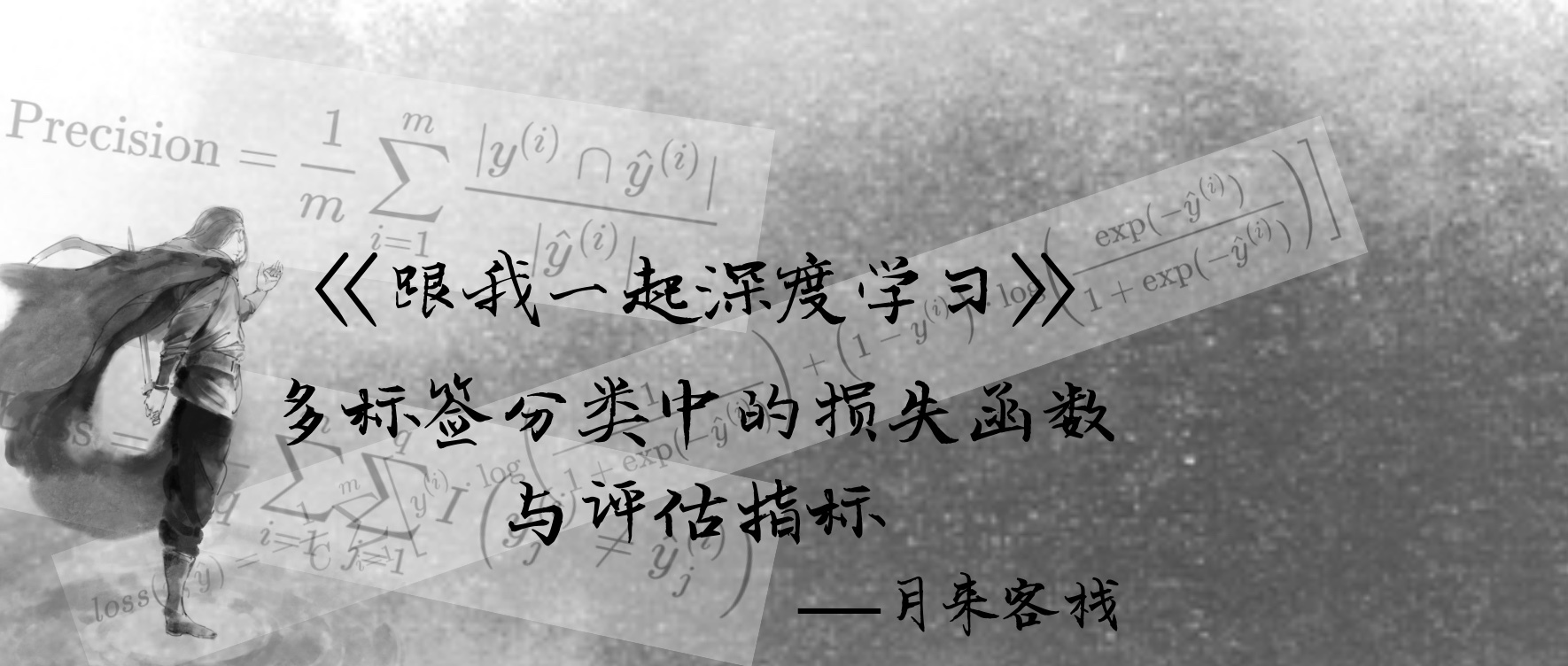# 多标签分类中的损失函数与评价指标### 2 方法一

#### 2.1 numpy实现：

def sigmoid(z):    return 1 / (1 + np.exp(-z))def compute_loss_v1(y_true, y_pred):    t_loss = y_true * np.log(sigmoid(y_pred)) + \             (1 - y_true) * np.log(1 - sigmoid(y_pred))  # [batch_size,num_class]    loss = t_loss.mean(axis=-1)  # 得到每个样本的损失值, 这里可以是    return -loss.mean()  # 返回整体样本的损失均值（或其他）if __name__ == '__main__':    y_true = np.array([[1, 1, 0, 0], [0, 1, 0, 1]])    y_pred = np.array([[0.2, 0.5, 0, 0], [0.1, 0.5, 0, 0.8]])    print(compute_loss_v1(y_true, y_pred)) # 0.5926

#### 2.2 TensorFlow实现

Tensorflow 1.x中，可以通过tf.nn模块下的sigmoid_cross_entropy_with_logits方法进行调用：

def sigmoid_cross_entropy_with_logits(labels, logits):    loss = tf.nn.sigmoid_cross_entropy_with_logits(labels=labels, logits=logits)    loss = tf.reduce_mean(loss, axis=-1)    return tf.reduce_mean(loss)if __name__ == '__main__':    y_true = tf.constant([[1, 1, 0, 0], [0, 1, 0, 1]],dtype=tf.float16)    y_pred = tf.constant([[0.2, 0.5, 0, 0], [0.1, 0.5, 0, 0.8]],dtype=tf.float16)    with tf.Session() as sess:        loss = sess.run(sigmoid_cross_entropy_with_logits(y_true,y_pred))        print(loss) # 0.5926

def prediction(logits, K):      y_pred = np.argsort(-logits, axis=-1)[:,:K]    print("预测标签：",y_pred)    p = np.vstack([logits[r,c] for r,c in enumerate(y_pred)])    print("预测概率：",p)prediction(y_pred,2)#####预测标签：[[1 0] [3 1]]预测概率： [[0.5 0.2] [0.8 0.5]]

#### 2.3 Pytorch实现

Pytorch中，可以通过torch.nn模块中的MultiLabelSoftMarginLoss类来完成损失的计算：

if __name__ == '__main__':    y_true = torch.tensor([[1, 1, 0, 0], [0, 1, 0, 1]],dtype=torch.int16)    y_pred = torch.tensor([[0.2, 0.5, 0, 0], [0.1, 0.5, 0, 0.8]],dtype=torch.float32)    loss = nn.MultiLabelSoftMarginLoss(reduction='mean')    print(loss(y_pred, y_true)) #0.5926

### 3 方法二

y_true = np.array([[1, 1, 0, 0], [0, 1, 0, 1.]])y_pred = np.array([[0.2, 0.5, 0.1, 0], [0.1, 0.5, 0, 0.8]])

[[0.24549354 0.33138161 0.22213174 0.20099311] [0.18482871 0.27573204 0.16723993 0.37219932]]

#### 3.1 numpy实现：

def softmax(x):    s = np.exp(x)    return s / np.sum(s, axis=-1, keepdims=True)def compute_loss_v2(logits, y):    logits = softmax(logits)    print(logits)    c = -(y * np.log(logits)).sum(axis=-1)  # 计算每一个样本的在各个标签上的损失和    return np.mean(c)  # 计算所有样本损失的平均值y_true = np.array([[1, 1, 0, 0], [0, 1, 0, 1.]])y_pred = np.array([[0.2, 0.5, 0.1, 0], [0.1, 0.5, 0, 0.8]])print(compute_loss_v2(y_pred, y_true))# 2.392

#### 3.2TensorFlow实现

Tensorflow 1.x中，可以通过tf.nn模块下的softmax_cross_entropy_with_logits_v2方法进行调用：

def softmax_cross_entropy_with_logits(labels, logits):    loss = tf.nn.softmax_cross_entropy_with_logits_v2(labels=labels, logits=logits)    return tf.reduce_mean(loss)y_true = tf.constant([[1, 1, 0, 0], [0, 1, 0, 1.]], dtype=tf.float16)y_pred = tf.constant([[0.2, 0.5, 0.1, 0], [0.1, 0.5, 0, 0.8]], dtype=tf.float16)with tf.Session() as sess:    loss = sess.run(softmax_cross_entropy_with_logits(y_true, y_pred))    print(loss)# 2.395 

#### 3.3 Pytorch实现

Pytorch中，笔者目前还没找到可以调用的相应模型，但是可以通过自己来编码实现：

def cross_entropy(logits, y):    s = torch.exp(logits)    logits = s / torch.sum(s, dim=1, keepdim=True)    c = -(y * torch.log(logits)).sum(dim=-1)    return torch.mean(c)y_true = torch.tensor([[1, 1, 0, 0], [0, 1, 0, 1.]])y_pred = torch.tensor([[0.2, 0.5, 0.1, 0], [0.1, 0.5, 0, 0.8]])loss = cross_entropy(y_pred,y_true)print(loss)# 2.392

### 4 评估指标

#### 4.1 不考虑部分正确的评估方法

(1) 绝对匹配率（Exact Match Ratio

 y_true = np.array([[0, 1, 0, 1],                       [0, 1, 1, 0],                       [1, 0, 1, 1]])y_pred = np.array([[0, 1, 1, 0],                       [0, 1, 1, 0],                       [0, 1, 0, 1]])

from sklearn.metrics import accuracy_scoreprint(accuracy_score(y_true,y_pred)) # 0.33333333

（2）0-1 损失

）。绝对准确率计算的是完全预测正确的样本占总样本数的比例，而 0-1 损失计算的是完全预测错误的样本占总样本的比例。因此对于上面的预测和真实结果，其 0-1 损失就应该为 0.667。计算公式如下：

sklearn中，可以通过sklearn.metrics模块中的zero_one_loss方法来完成计算，如下所示：

from sklearn.metrics import zero_one_lossprint(zero_one_loss(y_true,y_pred))# 0.66666

#### 4.2 考虑部分正确的评估方法

（1）准确率

。那么该样本对应的准确率就应该为：

 y_true = np.array([[0, 1, 0, 1],                       [0, 1, 1, 0],                       [1, 0, 1, 1]])y_pred = np.array([[0, 1, 1, 0],                       [0, 1, 1, 0],                       [0, 1, 0, 1]])

def Accuracy(y_true, y_pred):    count = 0    for i in range(y_true.shape):        p = sum(np.logical_and(y_true[i], y_pred[i]))        q = sum(np.logical_or(y_true[i], y_pred[i]))        count += p / q    return count / y_true.shapeprint(Accuracy(y_true, y_pred)) # 0.52777

（2）精确率

。那么该样本对应的精确率就应该为：

def Precision(y_true, y_pred):    count = 0    for i in range(y_true.shape):        if sum(y_pred[i]) == 0:            continue        count += sum(np.logical_and(y_true[i], y_pred[i])) / sum(y_pred[i])    return count / y_true.shapeprint(Precision(y_true, y_pred))# 0.6666

（3）召回率

 y_true = np.array([[0, 1, 0, 1],                       [0, 1, 1, 0],                       [1, 0, 1, 1]])y_pred = np.array([[0, 1, 1, 0],                       [0, 1, 1, 0],                       [0, 1, 0, 1]])

def Recall(y_true, y_pred):    count = 0    for i in range(y_true.shape):        if sum(y_true[i]) == 0:            continue        count += sum(np.logical_and(y_true[i], y_pred[i])) / sum(y_true[i])    return count / y_true.shapeprint(Recall(y_true, y_pred))# 0.6111

（4）

def F1Measure(y_true, y_pred):    count = 0    for i in range(y_true.shape):        if (sum(y_true[i]) == 0) and (sum(y_pred[i]) == 0):            continue        p = sum(np.logical_and(y_true[i], y_pred[i]))        q = sum(y_true[i]) + sum(y_pred[i])        count += (2 * p) / q    return count / y_true.shapeprint(F1Measure(y_true, y_pred))# 0.6333

from sklearn.metrics import precision_score, recall_score, f1_scoreprint(precision_score(y_true=y_true, y_pred=y_pred, average='samples'))# 0.6666print(recall_score(y_true=y_true, y_pred=y_pred, average='samples'))# 0.6111print(f1_score(y_true,y_pred,average='samples'))# 0.6333

（5）Hamming Loss

 y_true = np.array([[0, 1, 0, 1],                       [0, 1, 1, 0],                       [1, 0, 1, 1]])y_pred = np.array([[0, 1, 1, 0],                       [0, 1, 1, 0],                       [0, 1, 0, 1]])

def Hamming_Loss(y_true, y_pred):    count = 0    for i in range(y_true.shape):        p = np.size(y_true[i] == y_pred[i])        q = np.count_nonzero(y_true[i] == y_pred[i])        count += p - q    return count / (y_true.shape * y_true.shape)print(Hamming_Loss(y_true, y_pred))# 0.4166

from sklearn.metrics import hamming_lossprint(hamming_loss(y_true, y_pred))# 0.4166

### 引用

 Scikit-learn: Machine Learning in Python, Pedregosa et al., JMLR 12, pp. 2825-2830, 2011.

 Sorower, Mohammad S.. “A Literature Survey on Algorithms for Multi-label Learning.” (2010).

 Godbole, S., & Sarawagi, S. Discriminative Methods for Multi-labeled Classification. Lecture Notes in Computer Science,(2004), 22–30.## 评论